# In a FlashLight is a form of radiant energy.

## Forms of energy

There are many different types of energy, which all fall into two primary formskinetic and potential. Energy can transform from one type to another, but it can never be destroyed or created.

# Burning Questions

## What are the different types of energy?

Types of energy can be categorised into two broad categories – kinetic energy (the energy of moving objects) and potential energy (energy that is stored). These are the two basic forms of energy. The different types of energy include thermal energy, radiant energy, chemical energy, nuclear energy, electrical energy, motion energy, sound energy, elastic energy and gravitational energy.

# Discover the different types of energy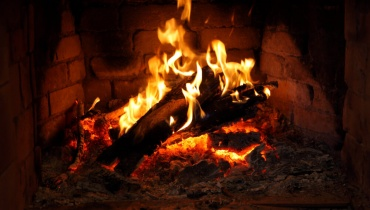### Thermal (Heat) Energy

Thermal energy is created from the vibration of atoms and molecules within substances. The faster they move, the more energy they possess and the hotter they become. Thermal energy is also called heat energy.

Let's go! >### Chemical Energy

Chemical energy is stored in the bonds of atoms and molecules – it is the energy that holds these particles together. Stored chemical energy is found in food, biomass, petroleum, and natural gas.

Let's go! >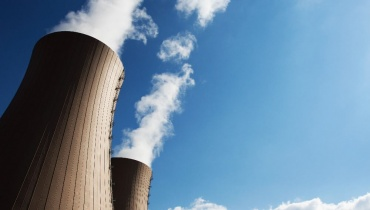### Nuclear Energy

Nuclear energy is stored in the nucleus of atoms. This energy is released when the nuclei are combined (fusion) or split apart (fission). Nuclear power plants split the nuclei of uranium atoms to produce electricity.

Let's go! >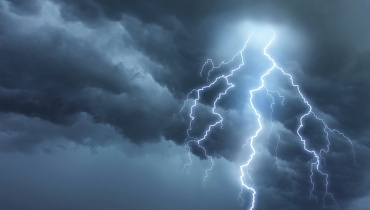### Electrical Energy

Electrical energy is the movement of electrons (the tiny particles that makeup atoms, along with protons and neutrons). Electrons that move through a wire are called electricity. Lightning is another example of electrical energy.

Let's go! >Also known as light energy or electromagnetic energy, radiant energy is a type of kinetic energy that travels in waves. Examples include the energy from the sun, x-rays, and radio waves.

Let's go! >### Light Energy

Light energy is a form of electromagnetic radiation. Light consists of photons, which are produced when an object's atoms heat up. Light travels in waves and is the only form of energy visible to the human eye.

Let's go! >### Motion Energy

Motion energy – or mechanical energy – is the energy stored in objects; as objects move faster, more energy is stored. Examples of motion energy include wind, a flowing river, a moving car, or a person running.

Let's go! >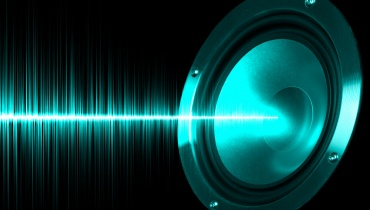### Sound Energy

Sound energy is the movement of energy through substances. It moves in waves and is produced when a force makes an object or substance vibrate. There is usually much less energy in sound than in other forms of energy.

Let's go! >### Elastic Energy

Elastic energy is a form of potential energy that is stored in an elastic object - such as a coiled spring or a stretched elastic band. Elastic objects store elastic energy when a force causes them to be stretched or squashed.

Let's go! >### Gravitational Energy

Gravitational energy is a form of potential energy. It is an energy associated with gravity or gravitational force – in other words, the energy held by an object when it is in a high position compared to a lower position.

Let's go! >

## What is the Law of Conservation of Energy?

While it might sound complex, the First Law of Conservation of energy simply states that energy can never be created or destroyed, but it can be transformed from one type to another.

# What Do You Mean?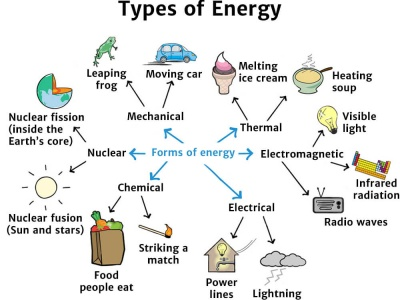Energy can be transformed from one form to another in different ways.

Kinetic energy is the energy of a moving object.

Potential energy is energy that is stored in an object or substance.

The Law of Conservation of energy is that energy can be transformed from one form to another, but can be neither created or destroyed.

Energy Transformations see diagram…

Notice that these energy transfer examples only show the useful energy transfers. However, car engines are also noisy (sound energy) and hot (thermal energy) and electric lamps also give out heat energy.

# Cool Facts

The word energy comes from the Greek word 'energeia', meaning 'activity.

# Cool Facts

The use of the word 'energy' dates all the way back to the 4th century BC.

# Speedy SummarySunlight energy is converted into the chemical energy of glucose.

Energy comes in many different types, which can be categorised into two basic formskinetic and potential. Energy can never be created or destroyed, but it can be transformed from one type of energy to another.

# Teacher's Toolkit

Take this to the classroom!